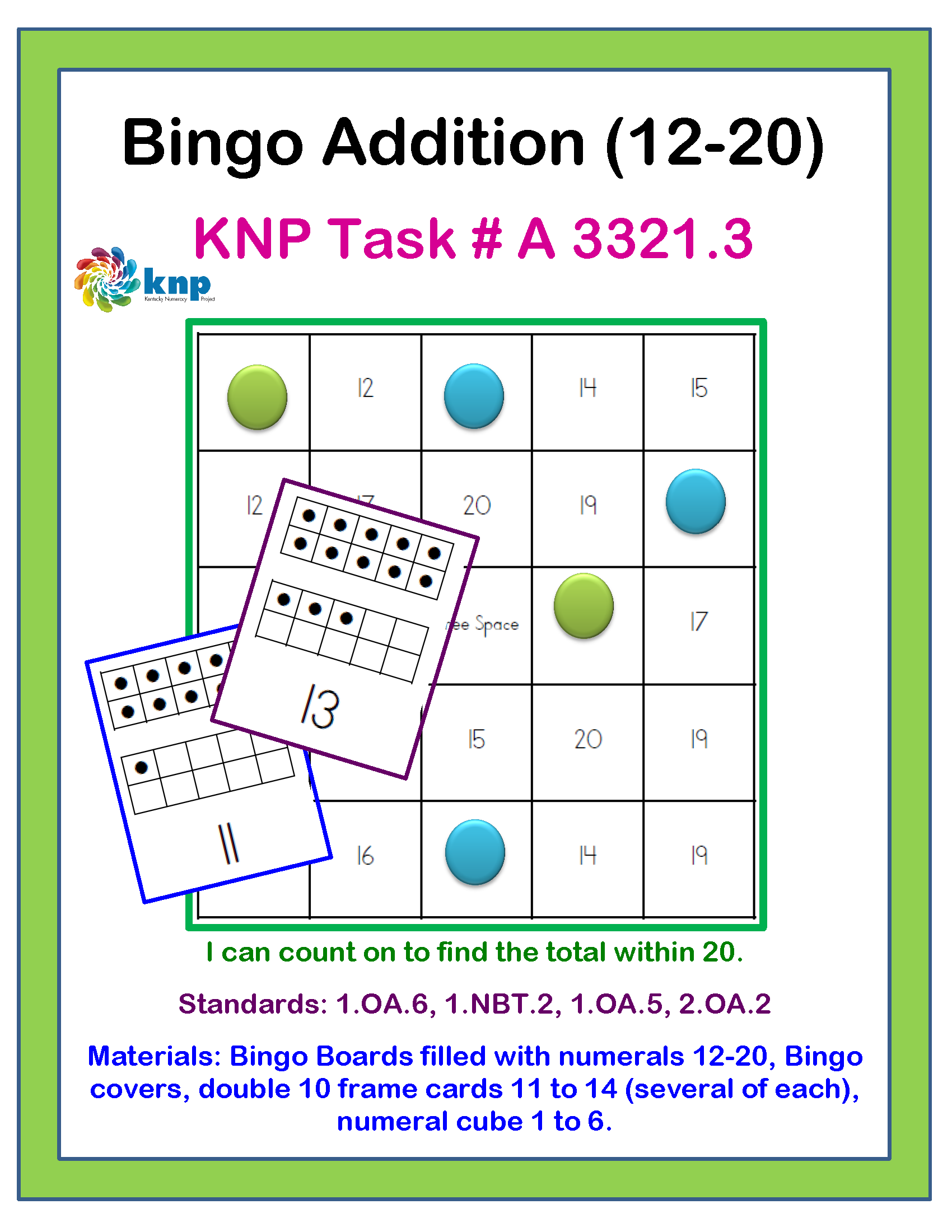# “Bingo Addition (12-20)”## Learning Target

I can count on to find the total within 20.

## Fluency Benchmark

2.OA.2 Fluently add and subtract within 20 using mental strategies. By end of Grade 2, know from memory all sums of two one-digit numbers.

## KAS(s)

1) 1.OA.5 Relate counting to addition and subtraction (e.g., by counting on 2 to add 2).   2) 1.NBT.2 Understand that the two digits of a two-digit number represent amounts of tens and ones. Understand the following as special cases: a. 10 can be thought of as a bundle of ten ones - called a "ten." b. The numbers from 11 to 19 are composed of a ten and one, two, three, four, five, six, seven, eight, or nine ones. c. The numbers 10, 20, 30, 40, 50, 60, 70, 80, 90 refer to one, two, three, four, five, six, seven, eight, or nine tens (and 0 ones).   3) 1.OA.6 Add and subtract within 20, demonstrating fluency for addition and subtraction within 10. Use strategies such as counting on; making ten (e.g., 8 + 6 = 8 + 2 + 4 = 10 + 4 = 14); decomposing a number leading to a ten (e.g., 13 - 4 = 13 - 3 - 1 = 10 - 1 = 9); using the relationship between addition and subtraction (e.g., knowing that 8 + 4 = 12, one knows 12 - 8 = 4); and creating equivalent but easier or known sums (e.g., adding 6 + 7 by creating the known equivalent 6 + 6 + 1 = 12 + 1 = 13).

KNPIG # A 3321.3

## Lesson Plan## Student Instructions## Printables## Family Activity## Would You Like to See More?

If you found this activity helpful and would like to see more, just register to gain access to the full KNP Intervention Guide.